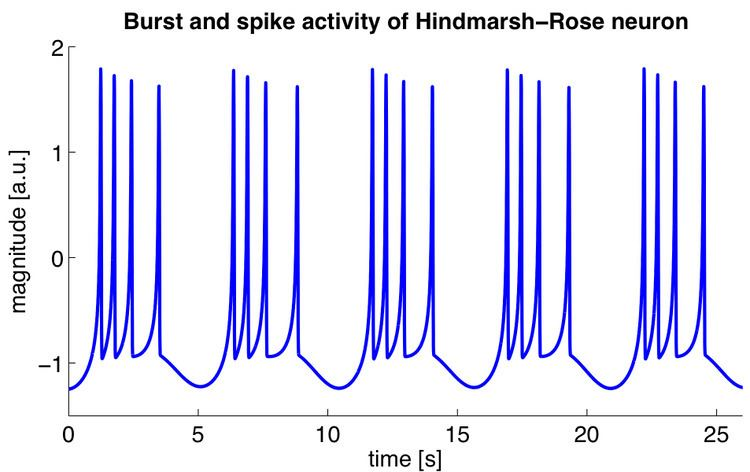# Hindmarsh–Rose model

Updated on
Covid-19The Hindmarsh–Rose model of neuronal activity is aimed to study the spiking-bursting behavior of the membrane potential observed in experiments made with a single neuron. The relevant variable is the membrane potential, x(t), which is written in dimensionless units. There are two more variables, y(t) and z(t), which take into account the transport of ions across the membrane through the ion channels. The transport of sodium and potassium ions is made through fast ion channels and its rate is measured by y(t), which is called the spiking variable. The transport of other ions is made through slow channels, and is taken into account through z(t), which is called the bursting variable. Then, the Hindmarsh–Rose model has the mathematical form of a system of three nonlinear ordinary differential equations on the dimensionless dynamical variables x(t), y(t), and z(t). They read:

d x d t = y + ϕ ( x ) z + I , d y d t = ψ ( x ) y , d z d t = r [ s ( x x R ) z ] ,

where

ϕ ( x ) = a x 3 + b x 2 , ψ ( x ) = c d x 2 .

The model has eight parameters: a, b, c, d, r, s, xR and I. It is common to fix some of them and let the others be control parameters. Usually the parameter I, which means the current that enters the neuron, is taken as a control parameter. Other control parameters used often in the literature are a, b, c, d, or r, the first four modeling the working of the fast ion channels and the last one the slow ion channels, respectively. Frequently, the parameters held fixed are s = 4 and xR = -8/5. When a, b, c, d are fixed the values given are a = 1, b = 3, c = 1, and d = 5. The parameter r is something of the order of 10−3, and I ranges between −10 and 10.

The third state equation:

d z d t = r [ s ( x x R ) z ] ,

allows a great variety of dynamic behaviors of the membrane potential, described by variable x, including unpredictable behavior, which is referred to as chaotic dynamics. This makes the Hindmarsh–Rose model relatively simple and provides a good qualitative description of the many different patterns that are observed empirically.

Similar Topics
The Missionary